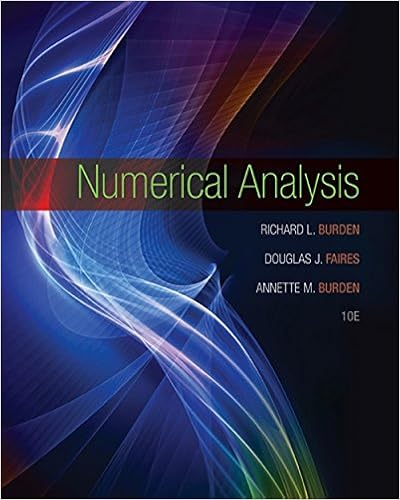# Ans a water is the limiting reagent b 950 g of h 2 s

• Test Prep
• 19
• 91% (33) 30 out of 33 people found this document helpful

This preview shows page 17 - 19 out of 19 pages.

##### We have textbook solutions for you!
The document you are viewing contains questions related to this textbook.The document you are viewing contains questions related to this textbook.
Chapter 6 / Exercise 11
Numerical Analysis
Burden/FairesExpert Verified
Ans:a. Water is the limiting reagent.b. 9.50 g of H2Sc. 1.11 g Al2S3remains.
Difficulty: M94.The insecticide DDT was formerly in widespread use, but now it is severely restricted owing to its adverse environmental effects. It is prepared as follows:C2HCl3O + 2C6H5Cl C14H9Cl5+ Hchloral chlorobenzene DDTIf 10.00 g of chloral were reacted with 10.00 g of chlorobenzenea. what is the maximum amount (mol) of DDT which could be formed?b. what is the limiting reagent?c. what is the % yield, if 12.15 g of DDT is produced?2O
Difficulty: H95.You are provided with a 250 mL volumetric flask, deionized water, and solid NaOH. How much NaOH should be weighed out in order to make 250. mL of 0.100 solution?M
Difficulty: E96.A solution of methanol (CH4O) in water has a concentration of 0.200 M. What mass of methanol, in grams, is present in 0.150 liters of this solution?
Page 46
##### We have textbook solutions for you!
The document you are viewing contains questions related to this textbook.The document you are viewing contains questions related to this textbook.
Chapter 6 / Exercise 11
Numerical Analysis
Burden/FairesExpert Verified
Chapter 3: Stoichiometry of Formulas and EquationsDifficulty: E97.a. A solution of common salt, NaCl, in water has a concentration of 0.0921 M. Calculatethe number of moles of NaCl contained in 50.0 mL of this solution.b. If, instead, an NaCl solution is prepared by dissolving 10.0 g of solid NaCl in enough water to make 250. mL of solution, what is the molarity?Ans:a. 0.00461 gb. 0.684 MDifficulty: E98.Aluminum metal dissolved in hydrochloric acid as follows:2Al(s) + 6HCl(aq) 2AlCl3(aq) + 3H2a. What is the minimum volume of 6.0 MHCl(aq) needed to completely dissolve 3.20 g of aluminum in this reaction?b. What mass of AlCl3 would be produced by complete reaction of 3.20 g of aluminum?(g)Ans:a. 59.3 mLb. 15.8 gDifficulty: M99.One mole of O2has a mass of 16.0 g.
Difficulty: E100.One mole of methane (CH4) contains a total of 3 1024atoms.
Difficulty: E101.The formula CH3O0.5is an example of an empirical formula.
Difficulty: E102.In combustion analysis, the carbon and hydrogen contents of a substance are determinedfrom the CO2and H2O, respectively, which are collected in the absorbers.
Difficulty: E103.In combustion analysis, the oxygen content of a substance is equal to the total oxygen inthe CO2and H2O collected in the absorbers.
Difficulty: M104.Constitutional (structural) isomers have the same empirical formula but different molecular formulas.
Difficulty: M105.Constitutional (structural) isomers have the same molecular formula but different structural formulas.
Difficulty: MPage 47
Chapter 3: Stoichiometry of Formulas and Equations106.In a correctly balanced equation, the number of reactant molecules must equal the number of product molecules.
Difficulty: E107.The correct method for preparing one liter of a 1.0 Msolution of X is to dissolve exactlyone mole of X in exactly one liter of water.
•••